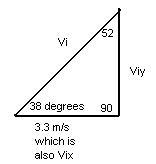# Need help on projectile

need help on projectile!!

## Homework Statement

A projectile is fired at an angle of 38 degrees with respect to the x axis and with an initial velocity in the x direction of 3.3 m/sec. (gravity=10m/sec^2)

a)whats the initial velocity of the projectile?

## Homework Equations

a) i think Vy=Vi(sin38 degrees)
and a^2+b^2=c^2

## The Attempt at a Solution

I first want to get the Vy by using: Vy=Vi(sin theta)
so, Vy=3.3m/sec(sin 38 degrees)
Vy=2.03 m/sec

now, (2.03)^2 + (3.3)^2= r^2
so r or the initial velocity of the projectile =3.87 m/s ????????

## Answers and Replies

OlderDan
Science Advisor
Homework Helper
Your reasoning is circular and incorrect. First you are using Vi to find Vy, and then you are using Vy and Vx to find what you called r, which is in fact Vi. You are not getting r = Vi because you did not know Vi when you used it to find Vy. What you are given in the problem is Vx and a launch angle. How do you find Vi from the given information?

let me try again, with the right variables and numbers:Viy=Vi/(tan theta)
Viy=3.3 m/sec / (tan 52 degrees)
Viy=2.58 m/sec

so then i do the phythagorem (sp?) theorm:
Vix+Viy=Vi
(3.3^2)+(2.58^2)=Vi
4.19 m/sec=Vi !!!!!!!!?!??!?!

i believe thats correct

cristo
Staff Emeritus
Science Advisor
let me try again, with the right variables and numbers:Viy=Vi/(tan theta)
Viy=3.3 m/sec / (tan 52 degrees)
Viy=2.58 m/sec

so then i do the phythagorem (sp?) theorm:
Vix+Viy=Vi
(3.3^2)+(2.58^2)=Vi

4.19 m/sec=Vi
i believe thats correct

Your answer is correct. However the lines in bold should read Vi2=Vix2+Viy2
Vi=sqrt{(3.3^2)+(2.58^2)}.

The Theorem to which you are referring is the Pythagorean Theorem (named after Pythagoras).